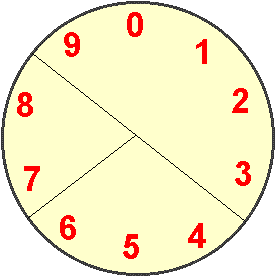#### You may also like### Prompt Cards

These two group activities use mathematical reasoning - one is numerical, one geometric.### Consecutive Numbers

An investigation involving adding and subtracting sets of consecutive numbers. Lots to find out, lots to explore.### Exploring Wild & Wonderful Number Patterns

EWWNP means Exploring Wild and Wonderful Number Patterns Created by Yourself! Investigate what happens if we create number patterns using some simple rules.

# Dividing a Cake

##### Age 7 to 11Challenge Level

Again, we received lots of solutions to this problem, but not many of you answered everything!

Alice, Gwen, Amber and Emma from The Downs C.E.P. School began by trying to divide the cake into equal sized pieces. They described how they soon found that the numbers didn't add up to the same total. They discovered another strategy which Serena and Philippa at Brighton College Prep School explain well:

Step $1$
Add up all the numbers on the cake. They should equal $45$.

Step $2$
Divide the number by 3 because that is how many pieces of cake they want.

Step $3$
Add up three sets of numbers that equal $1$5. You should have:
i) $9 + 0 + 1 + 2 + 3$
ii) $6 + 5 + 4$
iii) $8 + 7$

Many of you used this idea including Lewis and Emma from St Mary's RC School and Anders at Bentley Park College.
Jennifer from Crownfield Junior School tells us exactly how she did it:

At first I had no idea how to work it out. I was sitting there telling my mum every thought or question I had about it, and then it came to me. I went online to the Nrich website to find a clue. Once I had answered the clue, which was a question, I knew exactly how to work it out.

The answer was $45$, so as we had to cut the cake into three pieces. I divided $45$ by three. My answer was $15$. Therefore each piece would have to add up to $15$. I sat there making $15$ and finally I got it. I wasn't $100$% sure so I checked with my mum. Fortunately she told me I was correct.

I have only one piece of advice for anyone attempting to solve this problem that is stay calm and tell someone every question or thought you have about the problem.

Many more of you sent correct solutions like Sam from Congerstone Primary School.
Cansu, Gur, Burak, Koray and Kaan from Mef School in Turkey sent us the answer pictorially:However, only Daniel R (who hasn't given us his age or school); Alice, Gwen, Amber and Emma from The Downs C.E.P. School; Serena and Philippa at Brighton College Prep School and Daniel working at home in St Albans managed to work out what fraction of the whole cake each piece was.

Serena and Philippa explained how they did it:

Add up how many numbers there are = $10$

There are $5$ numbers on the first piece of cake = ${5\over10}$ (or ${1\over2}$)

There are $3$ numbers on the second piece of cake = ${3\over10}$

There are $2$ numbers on the third piece of cake = ${2\over10}$ (or ${1\over5}$)